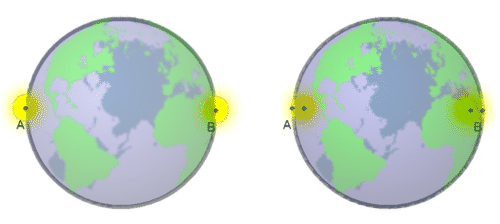# 19.2: A Few Extra Details

•• Contributed by CK12
• CK12

The word “about" was used here more than once. For instance, the orbital period of the Moon was stated to be “about" one month. In fact, the length of the “lunar month" from one new Moon to the next (or from one full Moon to the next) is 29.53 days, but the Moon's orbital period is actually 2.21 days shorter (this is discussed in the section on the calendar (See the chapter “The Calendar").

Viewed from Earth, a “new Moon" (occurring between the time a thin crescent is last seen before sunrise and the time one is seen shortly after sunset) happens when the Moon in its apparent motion around the sky overtakes the Sun. However, by the time of the next new Moon, the Sun's position in the sky has already shifted. If the Sun takes 12 months to go around the sky (or around the ecliptic, or around the zodiac), then in one month it completes 1/12 of its circuit. The Moon must therefore complete [1+(1/12)] circuits to catch up with the Sun again, and the lunar month (“synodic period") is about 1/12 of a month longer than the actual period of 27.32 days.

Also, the Earth's shadow has only approximately the width of $$2r$$. It would have very nearly a width of $$2r$$ if the Sun were a point-like light source (exactly that width if it were infinitely far away). Actually, however, the Sun is large enough to appear as a disk which covers about half a degree of the sky. As a result, the Earth's shadow is not a cylinder but a gradually narrowing cone, and at the Moon's distance it is already about 25% narrower than $$2r$$.

Here is another way of looking at the same process. Suppose we observe the eclipse from the Moon. Seen from there, the Earth moves from east to west --- from A to B in the drawing, assuming the eclipse is of greatest length (i.e. the middle of the Earth passes in front of the Sun).Figure $$\PageIndex{1}$$: Earth blocking the Sun during a lunar eclipse (not to scale).

The eclipse begins when the last bit of the western edge of the Sun passes point A (right of the drawing shown) and ends when the first bit of the eastern edge of the Sun pokes out at point B. That takes less time than it takes for the center of the Sun to pass from A to B (left drawing) which would be the duration of the eclipse if the Sun were a tiny point source, located at its middle.